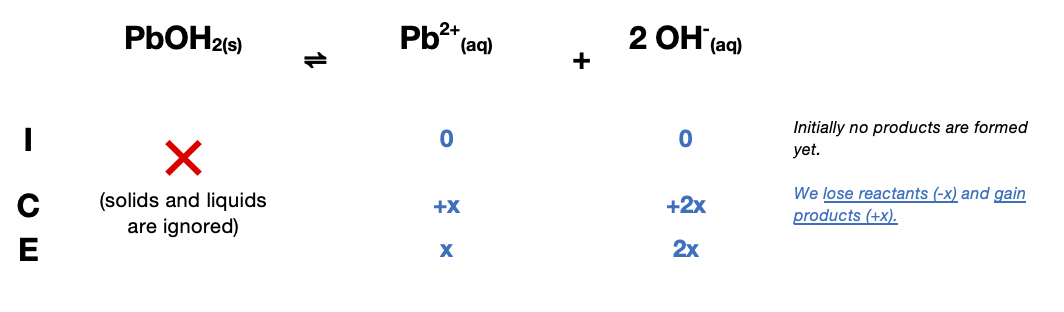# Problem: Calculate the concentration of Pb2+ in the following.a. a saturated solution of Pb(OH)2, Ksp = 1.2 X 10 -15

###### FREE Expert Solution$\overline{){{\mathbf{K}}}_{{\mathbf{sp}}}{\mathbf{=}}\frac{\mathbf{products}}{\overline{)\mathbf{reactants}}}{\mathbf{=}}\mathbf{\left[}{\mathbf{Pb}}^{\mathbf{2}\mathbf{+}}\mathbf{\right]}{\mathbf{\left[}{\mathbf{OH}}^{\mathbf{-}}\mathbf{\right]}}^{{\mathbf{2}}}}$

${\mathbf{1}}{\mathbf{.}}{\mathbf{2}}{\mathbf{×}}{{\mathbf{10}}}^{\mathbf{-}\mathbf{15}}{\mathbf{=}}\left(x\right){\left(2x\right)}^{{\mathbf{2}}}\phantom{\rule{0ex}{0ex}}\phantom{\rule{0ex}{0ex}}{\mathbf{1}}{\mathbf{.}}{\mathbf{2}}{\mathbf{×}}{{\mathbf{10}}}^{\mathbf{-}\mathbf{15}}{\mathbf{=}}\mathbf{\left(}\mathbf{x}\mathbf{\right)}\left(4{x}^{2}\right)\phantom{\rule{0ex}{0ex}}\phantom{\rule{0ex}{0ex}}\frac{\mathbf{1}\mathbf{.}\mathbf{2}\mathbf{×}{\mathbf{10}}^{\mathbf{-}\mathbf{15}}}{\mathbf{4}}{\mathbf{=}}\frac{\overline{)\mathbf{4}}{\mathbf{x}}^{\mathbf{3}}}{\overline{)\mathbf{4}}}\phantom{\rule{0ex}{0ex}}\phantom{\rule{0ex}{0ex}}\sqrt{{\mathbf{x}}^{\mathbf{3}}}{\mathbf{=}}\sqrt[\mathbf{3}]{\frac{\mathbf{1}\mathbf{.}\mathbf{2}\mathbf{×}{\mathbf{10}}^{\mathbf{-}\mathbf{15}}}{\mathbf{4}}}$###### Problem Details

Calculate the concentration of Pb2+ in the following.

a. a saturated solution of Pb(OH)2, Ksp = 1.2 X 10 -15